# Direct Labor: Standard Cost, Rate Variance, Efficiency Variance

Direct labor refers to the work done by employees who work directly on the goods being produced. (Indirect labor refers to the employees who work in the production area, but do not work directly on the products. An example of indirect labor is the employees who set up or maintain the equipment.)

Unlike direct materials (which are obtained prior to being used) direct labor is obtained and used at the same time. This means that for the given good output, we can compute the following at the same time (when goods are produced):

• Standard direct labor cost
• Direct labor rate variance
• Direct labor efficiency variance

### January 2022

Let's begin by determining the standard cost of direct labor for the good output produced in January 2022: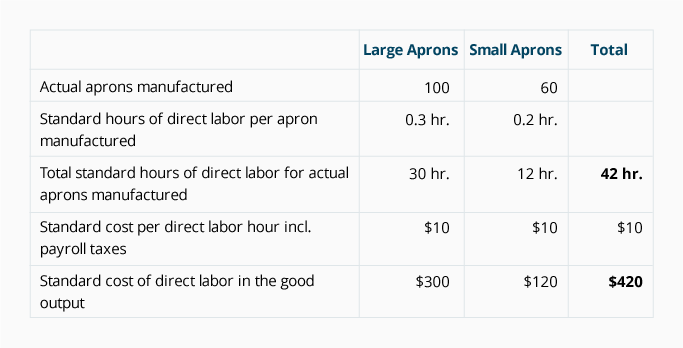Assuming that the actual direct labor in January adds up to 50 hours and the actual hourly rate of pay (including payroll taxes) is \$9 per hour, our analysis will look like this:

Direct Labor Variance Analysis for January 2022: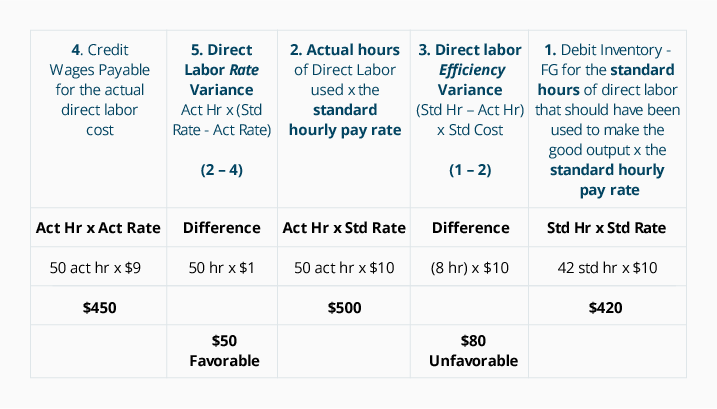In January, the direct labor efficiency variance (#3 in the above analysis) is unfavorable because the company actually used 50 hours of direct labor, which is 8 hours more than the standard quantity of 42 hours allowed for the good output. The additional 8 hours multiplied by the standard rate of \$10 results in an unfavorable direct labor efficiency variance of \$80. (The direct labor efficiency variance could also be referred to as the direct labor quantity variance or usage variance.)

Note that DenimWorks paid \$9 per hour for labor when the standard rate is \$10 per hour. This \$1 difference is multiplied by the 50 actual hours, resulting in a \$50 favorable direct labor rate variance. (The direct labor rate variance could be referred to as the direct labor price variance.)

The journal entry for the direct labor used in the January production is: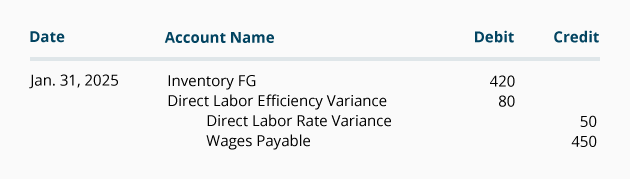### February 2022

In February DenimWorks manufactured 200 large aprons and 100 small aprons. The standard cost of direct labor and the variances for the February 2022 output is computed next.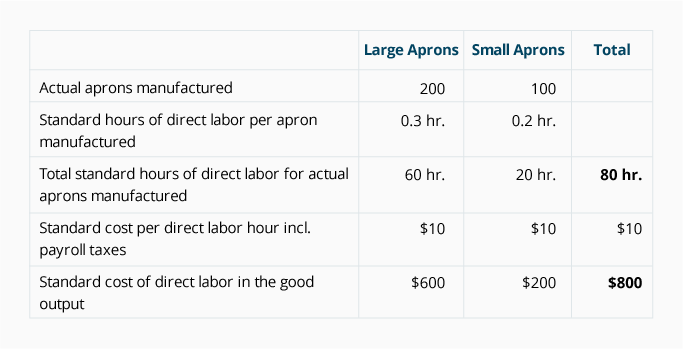If we assume that the actual labor hours in February add up to 75 and the hourly rate of pay (including payroll taxes) is \$11 per hour, the total equals \$825. The analysis for February 2022 looks like this:

Direct Labor Variance Analysis for February 2022: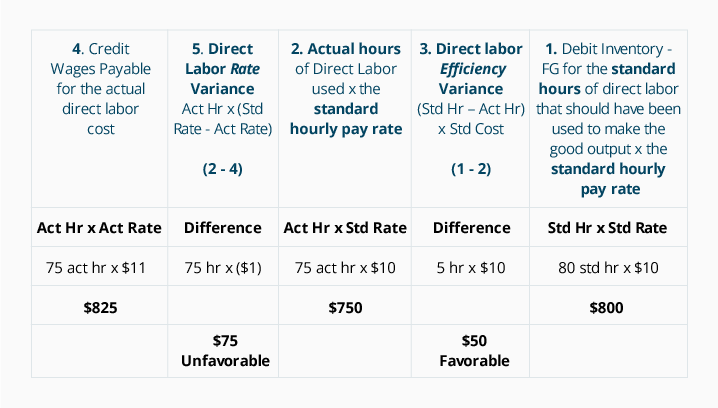Notice that for February's good output, the total actual labor costs amounted to \$825 and the total standard cost of direct labor amounted to \$800. This unfavorable difference of \$25 agrees to the sum of the two labor variances: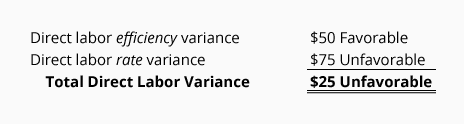The journal entry for the direct labor used in the February production is: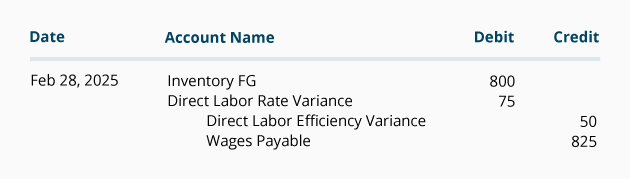Later in Part 6 we will discuss what to do with the balances in the direct labor variance accounts under the heading What To Do With Variance Amounts.